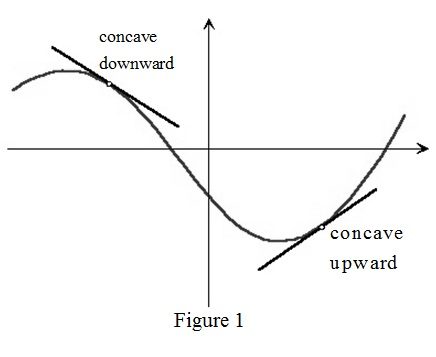# To state: The increasing / decreasing test.### Single Variable Calculus: Concepts...

4th Edition
James Stewart
Publisher: Cengage Learning
ISBN: 9781337687805### Single Variable Calculus: Concepts...

4th Edition
James Stewart
Publisher: Cengage Learning
ISBN: 9781337687805

#### Solutions

Chapter 4, Problem 5RCC

(a)

To determine

## To state: The increasing / decreasing test.

Expert Solution

### Explanation of Solution

The Increasing/decreasing test:

1) If f(x)>0 on an interval then f is increasing on that interval.

2) If f(x)<0 on an interval then f is decreasing on that interval.

(b)

To determine

Expert Solution

### Explanation of Solution

If graph of a function f lies above all its tangents on interval I then f is known as concave upward over interval I.In Figure 1, it is shown that for a concave upward curve the tangent lies below the graph of the curve while a concave downward curve the tangent lies above the graph of the curve.

(c)

To determine

Expert Solution

### Explanation of Solution

The concavity test:

1) If f(x)>0 for all x in I then graph of f is concave upward on interval I.

2) If f(x)<0 for all x in I then graph of f is concave downward on interval I.

(d)

To determine

Expert Solution

### Explanation of Solution

Inflection point:

“A point P on a curve y=f(x) is called inflection point if f is continuous at P and the curve changes from concave upward to concave downward or vice versa”.

An inflection point is at (x,f(x)) for any value of x where the concavity changes.

### Have a homework question?

Subscribe to bartleby learn! Ask subject matter experts 30 homework questions each month. Plus, you’ll have access to millions of step-by-step textbook answers!